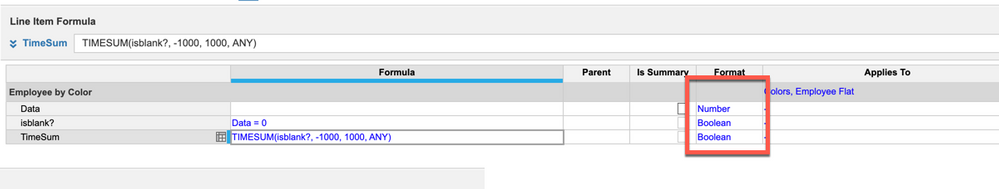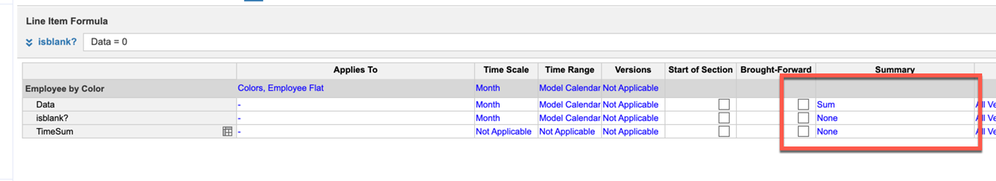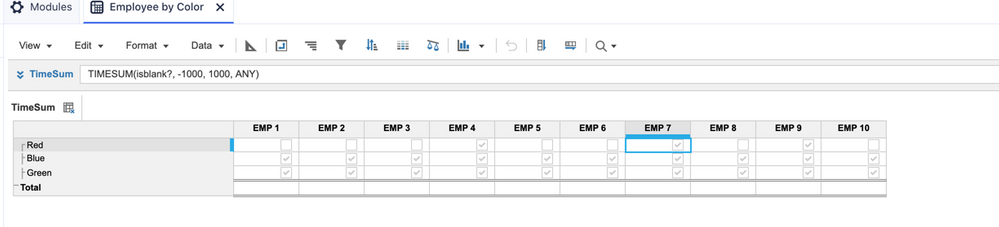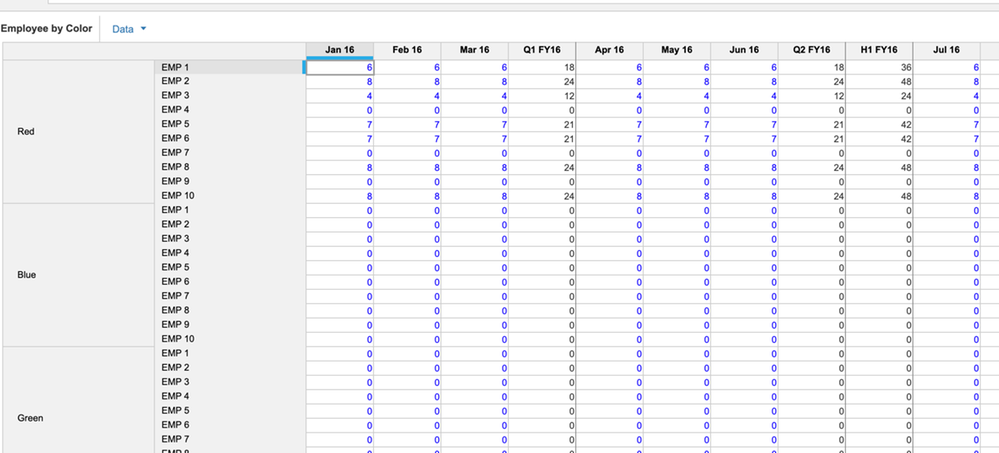# Applying Boolean formula from a time dimensioned to a non-time dimensioned modelHiya,

I have 2 modules:

Meldaliste Sales - 2 flat lists, time

System_Product_Filter - 2 flat lists

The flat lists in both modules are the same. I need to create a boolean formula in System_Product_Filter that says if there is a blank cell in Meldaliste Sales then false else true.

This formula isn't working because I have a time dimension in Meldaliste Sales, so the results pull through nothing in System_Product_Filter.

Any ideas would help.

Thanks

Hina 🙂

Tagged:

•First Check whether the time summary of the source module is not "None".

Then, assuming you have "All Periods" in time dimension, you can give the formula as isnotblank(MeldalisteSales[Select:Time.'All Periods']).

Hope this helps

•If you don't have "all Periods" in the time dimension, then you can use a TIMESUM formula with a blank "start period", which effectively sums all periods.  The formula would be: TIMESUM(Module with time.Amount) = 0

The only problem with this is that it effectively takes the sum of all periods, so if you have a -\$1000 in one period and then a +\$1000 in another period, then it treats it like a blank.  The overcome this, you could setup a 2nd line item in the module that includes time to dictate whether or not each cell has a value.  Maybe call this line item "check if blank".  So the formula would be: IF Amount <> 0 then 1 else 0.  Then, point that TIMESUM formula to the "check if blank" line item instead (so it would be TIMESUM(Module with time.'check if blank')).

Hope this helps!

Josh

•That way is fine, just please make sure the line item that has TimeSum() in it does not have Time in the Applies To.  And to get around your -1000 and 1000, you can use a boolean.Hope this helps,

Rob